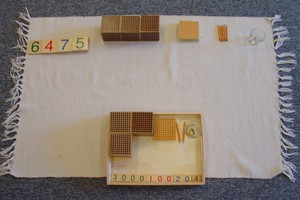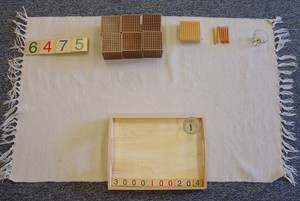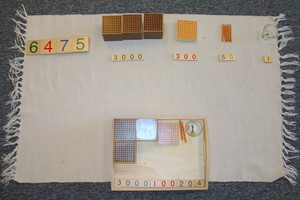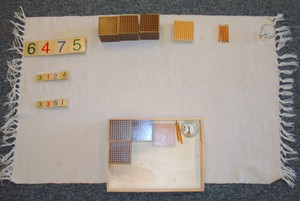# Static Subtraction With Golden Beads

Math - Arithmetical OperationsLevelPrimary
Age4.5 - 5
PrerequisitesBeads - Association of Symbol and Quantity
1 set of large number cards
2 sets of small number cards
1 tray for the child
1 small bowl for units

This activity teaches the child about subtraction.

## Presentation

1. The teacher should start the lesson with a large quantity of beads on the mat labeled with the large number cards.
The child should have a tray with small number cards showing a smaller number.
2. The teacher should point to the large quantity on the mat: "I have all these beads."
Then point to the number on the tray: "I am going to share this many with you."3. "How many units do you need?" Indicate the number card on the child's tray. Have the child take that many units.
Then do the same for the tens, the hundreds, and the thousands.4. Count and label the amount that is left on the mat.5. Stack the number cards and put in order as they would be in a subtraction problem. (In this case: 6475 - 3124 = 3351)
Point to the first number: "I had this many." Point to the second number: "Then you took this many." Point to the last number: "Now this is how many I have left. We just did subtraction."6. After the initial presentation, the child could do this work alone or with another child.